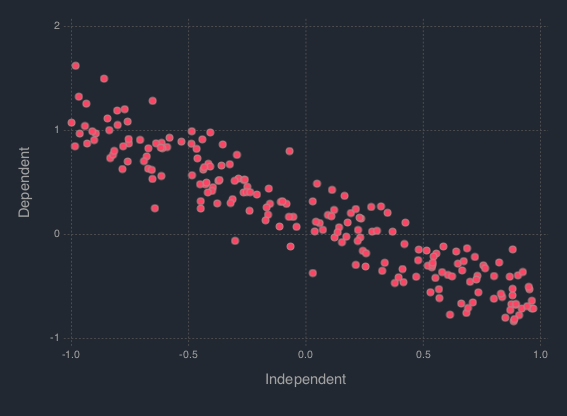# Hamiltonian Monte Carlo¶

Implementation of the Hamiltonian Monte Carlo sampler for Bayesian inference.

`HMC`(U::Function, K::Function, dU::Function, dK::Function, init_est::Array{Float64}, d::Int64)

Construct a `Sampler` object for Hamiltonian Monte Carlo sampling.

Arguments

• `U` : the potential energy or the negative log posterior of the parameter of interest.
• `K` : the kinetic energy or the negative exponential term of the log auxiliary distribution.
• `dU` : the gradient or first derivative of the potential energy `U`.
• `dK` : the gradient or first derivative of the kinetic energy `K`.
• `init_est` : the initial/starting value for the markov chain.
• `d` : the dimension of the posterior distribution.

Value

Returns a `HMC` type object.

Example

In order to illustrate the modeling, the data is simulated from a simple linear regression expectation function. That is the model is given by

```y_i = w_0 + w_1 x_i + e_i,   e_i ~ N(0, 1 / a)
```

To do so, let `B = [w_0, w_1]' = [.2, -.9]', a = 1 / 5`. Generate 200 hypothetical data:

```using DataFrames
using Distributions
using StochMCMC

srand(123);

# Define data parameters
w0 = -.3; w1 = -.5; stdev = 5.; a =  1 / stdev

# Generate Hypothetical Data
n = 200;
x = rand(Uniform(-1, 1), n);
A = [ones(length(x)) x];
B = [w0; w1];
f = A * B;
y = f + rand(Normal(0, a), n);

my_df = DataFrame(Independent = round(x, 4), Dependent = round(y, 4));
```

Next is to plot this data which can be done as follows:

```plot(my_df, x = :Independent, y = :Dependent)
```In order to proceed with the Bayesian inference, the parameters of the model is considered to be random modeled by a standard Gaussian distribution. That is, `B ~ N(0, I)`, where `0` is the zero vector. The likelihood of the data is given by,
```L(w|[x, y], b) = ∏_{i=1}^n N([x_i, y_i]|w, b)
```

Thus the posterior is given by,

```P(w|[x, y]) ∝ P(w)L(w|[x, y], b)
```

To start programming, define the probabilities

```"""
The log prior function is given by the following codes:
"""
function logprior(theta::Array{Float64}; mu::Array{Float64} = zero_vec, s::Array{Float64} = eye_mat)
w0_prior = log(pdf(Normal(mu[1, 1], s[1, 1]), theta))
w1_prior = log(pdf(Normal(mu[2, 1], s[2, 2]), theta))
w_prior = [w0_prior w1_prior]

return w_prior |> sum
end

"""
The log likelihood function is given by the following codes:
"""
function loglike(theta::Array{Float64}; alpha::Float64 = a, x::Array{Float64} = x, y::Array{Float64} = y)
yhat = theta + theta * x

likhood = Float64[]
for i in 1:length(yhat)
push!(likhood, log(pdf(Normal(yhat[i], alpha), y[i])))
end

return likhood |> sum
end

"""
The log posterior function is given by the following codes:
"""
function logpost(theta::Array{Float64})
loglike(theta, alpha = a, x = x, y = y) + logprior(theta, mu = zero_vec, s = eye_mat)
end
```

To start the estimation, define the necessary parameters

```# Hyperparameters
zero_vec = zeros(2)
eye_mat = eye(2)
```

Setup the necessary paramters including the gradients. The potential energy is the negative logposterior given by `U`, the gradient is `dU`; the kinetic energy is the standard Gaussian function given by `K`, with gradient `dK`. Thus,

```U(theta::Array{Float64}) = - logpost(theta);
K(p::Array{Float64}; Σ = eye(length(p))) = (p' * inv(Σ) * p) / 2;
function dU(theta::Array{Float64}; alpha::Float64 = a, b::Float64 = eye_mat[1, 1])
[-alpha * sum(y - (theta + theta * x));
-alpha * sum((y - (theta + theta * x)) .* x)] + b * theta
end
dK(p::AbstractArray{Float64}; Σ::Array{Float64} = eye(length(p))) = inv(Σ) * p;
```

Run the MCMC:

```srand(123);
HMC_object = HMC(U, K, dU, dK, zeros(2), 2);
chain2 = mcmc(HMC_object, leapfrog_params = Dict([:ɛ => .09, :τ => 20]), r = 10000);
```

Extract the estimate

```est2 = mapslices(mean, chain2[(burn_in + 1):thinning:end, :], );
est2
# 1×2 Array{Float64,2}:
#  -0.307151  -0.458954
```